##### Physics I Workbook For Dummies with Online PracticeThere’s often more than one force involved when you’re dragging a mass over a distance. Just think of the forces of friction and gravity for an object on an inclined plane. For example, take a look at the figure, where a couch is being dragged up an incline. If you’re applying force F, how much work is done as the couch is dragged up the incline?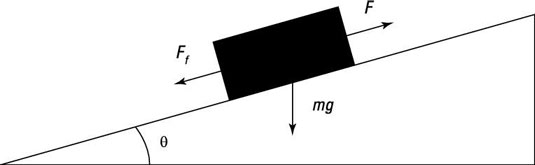Look at the forces involved in this scenario: There’s the force you apply (your pull), the force due to friction that resists your pull, and the force due to gravity that also resists your pull. While all these forces do work on the couch as it’s being pulled up the slope, the net work done on the couch is the product of the component of the net force parallel to the couch’s displacement multiplied by that displacement. In this situation, the net force and the displacement are in the same direction, so this simplifies to just net force multiplied by displacement.

In other words, you may do 100 J of work on the couch; friction, which is opposing you, may do –50 J (the work is negative because the force here is opposite to the direction traveled); and the force of gravity may do –30 J. That means that a net work of 20 J was done on the couch by all the forces (100 – 50 –30 = 20 J).

## Sample question

1. Suppose that the couch you’re dragging has a mass of 75.0 kg, and the angle of the ramp is 24.0 degrees. If the coefficient of kinetic friction is 0.170 and you’re pulling the couch 2.00 m with a force of 800.0 N, how much work is being done by the net force on the couch?

The correct answer is 774 J.

1. Identify the forces on the couch. You’re pulling with a force F in the direction of the ramp, so the component of your force in the direction of travel is exactly 800 N.

2. Look at the component of the force due to gravity downhill along the direction of the incline.

The figure tells you that this force is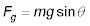(You can verify this by noting that Fg goes to mg as theta goes to 90 degrees and to 0 as theta goes to 0 degree.)

3. Calculate the force due to friction. That’s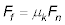where Fn is the normal force. The normal force in this scenario is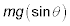so that makes the force due to friction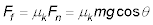4. Calculate the net force (upward along the incline):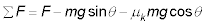5. Note that the forces due to friction and gravity are negative because they oppose the direction of travel.

6. Because all forces act along the direction of travel, the net work, W, is equal to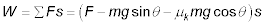7. Plug in the numbers: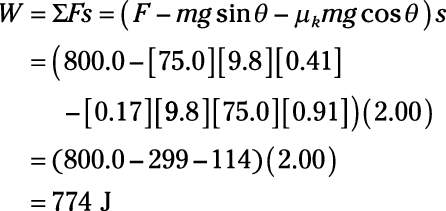## Practice questions

1. You’re pulling a chest of drawers, applying a force of 600.0 N parallel to the slope. The angle of the slope is 40.0 degrees. The chest has a mass of 40.0 kg, and there’s a coefficient of kinetic friction of 0.120.

How much net work do you do in pulling the chest of drawers 10.0 m up the inclined plane?

2. You’re applying a force of 800.0 N to the ground as you go up a ski slope on your new skis; this force is applied parallel to the slope (downhill).

Thus, the ground is necessarily pushing you with an equal force of 800.0 N in the opposite direction (uphill). If the slope is 22.0 degrees, the coefficient of kinetic friction is 0.050, and you have a mass of 80.0 kg, how much work does the ground do pushing you up that slope 500.0 m? How much net work is done?

Following are answers to the practice questions:

1. 3,120 J

1. Find the forces on the chest of drawers. You’re pulling with a force F in the direction of the ramp, so the component of your force in the direction of travel is 600.0 N.

2. Find the force due to gravity along the direction of the ramp. Keep in mind that this force will be going down the ramp.3. Find the force due to friction. That’swhere Fn is the normal force. The normal force here isso that makes the force due to friction4. Calculate the net force:5. Note that the force due to friction and gravity are negative because they oppose the direction of travel.

6. 5.The net work done is the total force along the direction of travel times the distance traveled. Because all forces act along the direction of travel, the net work, W, is7. Plug in the numbers: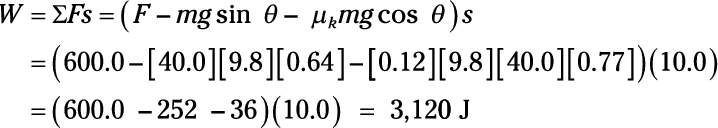2. The ground does 4.00 x 105 J of work. The net work is 2.35 x 105 J.

1. The ground is pushing you up the slope with a force of 800.0 N; this force goes along the direction of the slope.

2. The force due to gravity parallel to the slope isThis force is going down the slope.

3. The force due to friction iswhere Fn is the normal force. The normal force here is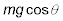so that makes the force due to friction:4. Calculate the net force:5. The net work done is the total force along the direction of travel times the distance traveled. Because all forces act along the direction of travel, the net work, Wnet, is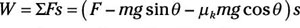6. Plug in the numbers to find the net work: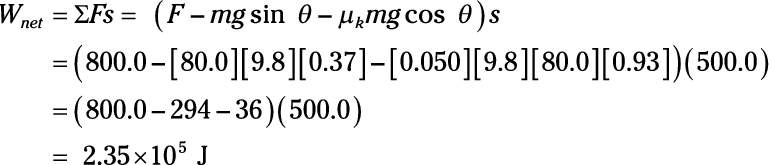7. The work done by the ground pushing on you is (800.0)(500.0) = 4.000 x 105 J.